Students can download Maths Chapter 4 Geometry Ex 4.6 Questions and Answers, Notes, Samacheer Kalvi 9th Maths Guide Pdf helps you to revise the complete Tamilnadu State Board New Syllabus, helps students complete homework assignments and to score high marks in board exams.

## Tamilnadu Samacheer Kalvi 9th Maths Solutions Chapter 4 Geometry Ex 4.6

Question 1.
Draw a triangle ABC, where AB = 8 cm, BC = 6 cm and ∠B = 70° and locate its circumcentre and draw the circumcircle.
Solution: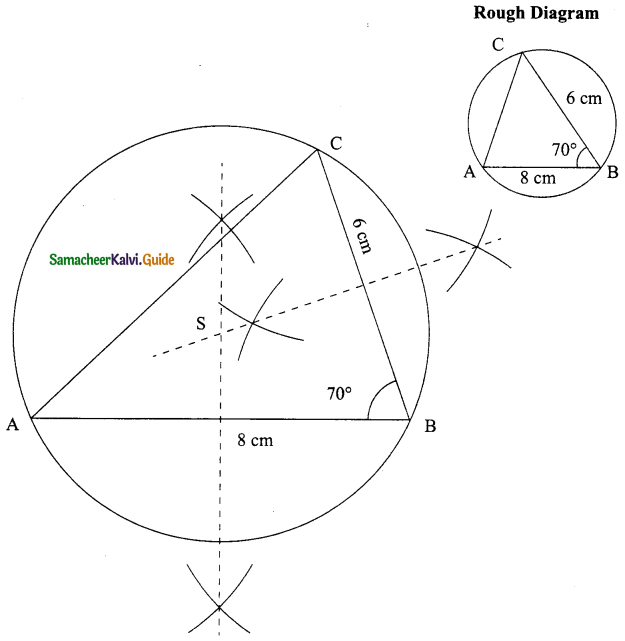Steps for construction:
Step 1: Draw the ΔABC with the given measures.
Step 2: Construct the perpendicular bisector of (AB and BC) any two sides and let them, meet at S which is the circumcenter.
Step 3: With S as centre and SA = SB = SC as radius draw the circumcircle to passes through A, B and C.Question 2.
Construct the right triangle PQR whose perpendicular sides are 4.5 cm and 6 cm. Also locate its circumcentre and draw circumcircle.
Solution: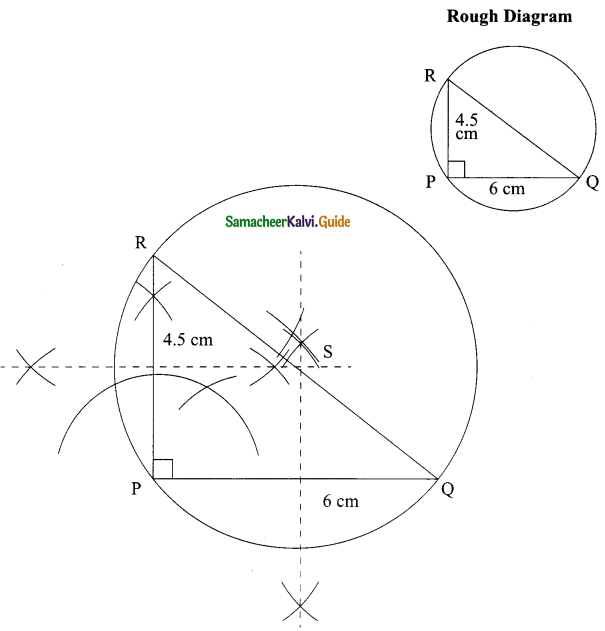Steps for construction:
Step 1: Draw the ΔPQR with the given measures.
Step 2: Construct the perpendicular bisector of (PQ and PR) any two sides and let them meet at S which is the circumcenter.
Step 3: With S as centre and SP = SQ = SR as radius draw the circumcircle to passes through P, Q and R.Question 3.
Construct ΔABC with AB = 5 cm ∠B = 100° and BC = 6 cm. Also locate its circumcentre draw circumcircle.
Solution: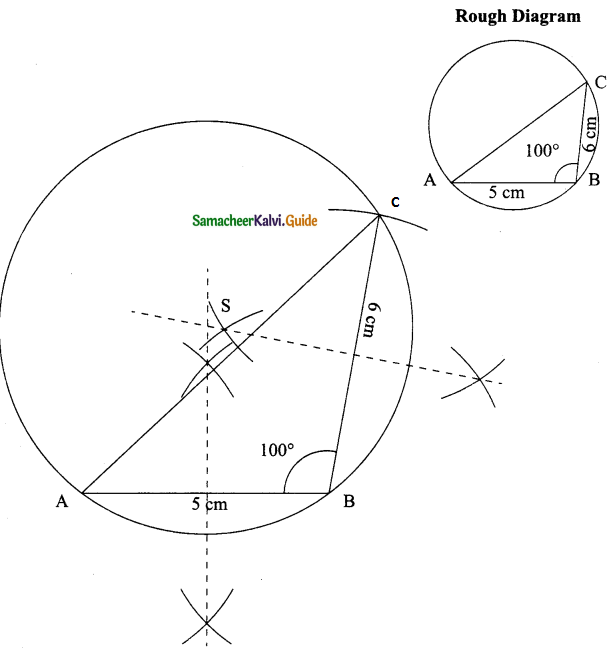Steps for construction:
Step 1: Draw the ΔABC with the given measures.
Step 2: Construct the perpendicular bisector of any two sides (AB and BC) and let them meet at S which is the circumcenter.
Step 3: With S as centre and SA = SB = SC as radius draw the circumcircle to passes through A, B and C.Question 4.
Construct an isosceles triangle PQR where PQ = PR and ∠Q = 50°, QR = 7cm. Also draw its circumcircle.
Solution: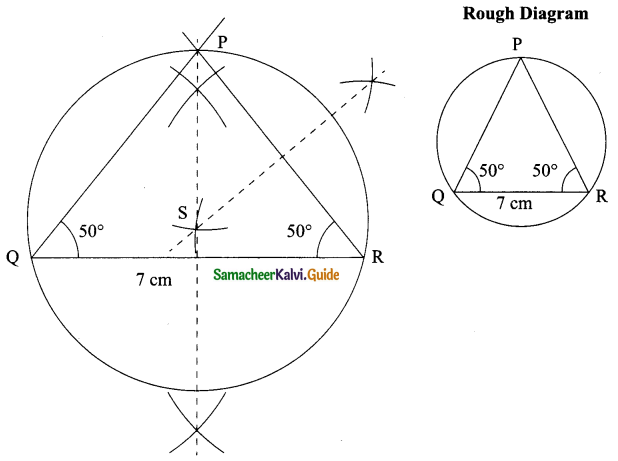Given PQ = PR
∴ ∠R = 50° (opposite angles are equal)
Steps for construction:
Step 1: Draw the ΔABC with the given measures.
Step 2: Construct the perpendicular bisector of any two sides (QR and PR) and let them meet at S. S is the circumcenter of ΔPQR.
Step 3: With S as centre SP = SQ = SR as radius. Draw the circumcircle.Question 5.
Draw an equilateral triangle of side 6.5 cm and locate its incentre. Also draw the incircle.
Solution: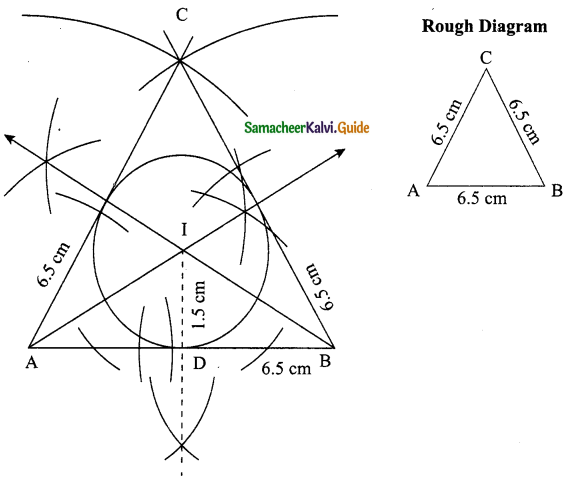Steps for construction:
Step 1: Draw the ΔABC with the each side measure 6.5 cm.
Step 2: Construct the angles bisectors of any two angles (A and B) and let them meet at I. Then I is the incentre of ΔABC.
Step 3: Draw perpendicular from I to any one of the side (AB) to meet AB at D.
Step 4: With I as centre and ID as radius draw the circle. This circle touches all the sides of the triangle internally.
Step 5: Measure of In-radius = 1.5 cm.Question 6.
Draw a right triangle whose hypotenuse is 10 cm and one of the legs is 8 cm. Locate its incentre and also draw the incircle
Solution: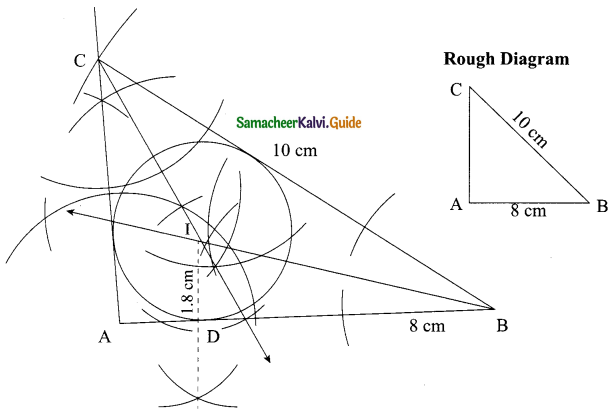Steps for construction:
Step 1: Draw the ΔABC with AB = 8cm, BC = 10 cm and ∠A = 90°.
Step 2: Construct the angle bisectors of any two angles (∠B and ∠C) and let them meet at I. Then I is the incentre of ΔABC.
Step 3: Draw perpendicular from I to any one of the side to meet AB at D.
Step 4: With I as centre and ID as radius draw the circle. This circle touches all the sides of the triangle internally.
Step 5: Measure of In-radius = 1.8 cm.Question 7.
Draw ΔABC given AB = 9 cm, ∠CAB = 115° and ∠ABC = 40°. Locate its incentre and also draw the incircle. (Note: You can check from the above examples that the incentre of any triangle is always in its interior).
Solution: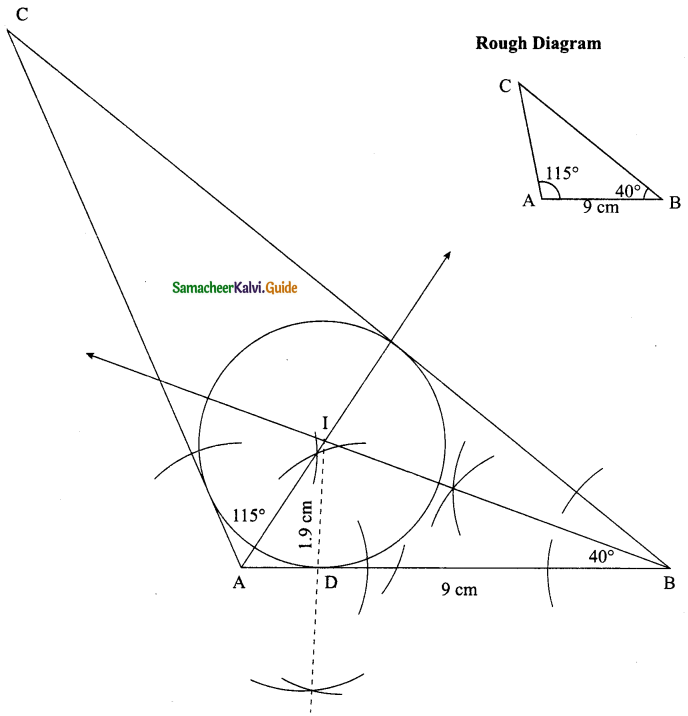Step 1: Draw the ΔABC with AB = 9cm, ∠B = 40° and ∠A = 115°.
Step 2: Construct the angle bisectors of any two angles (A and B) and let them meet at I. Then I is the incentre of ΔABC.
Step 3: Draw perpendicular from I to any one of the side (AB) to meet AB at D.
Step 4: With I as centre and ID as radius draw the circle. This circle touches all the sides of the triangle internally.
Step 5: Measure of In-radius = 2.7 cm.Question 8.
Construct ΔABC in which AB = BC = 6cm and ∠B = 80°. Locate its incentre and draw the incircle.
Solution: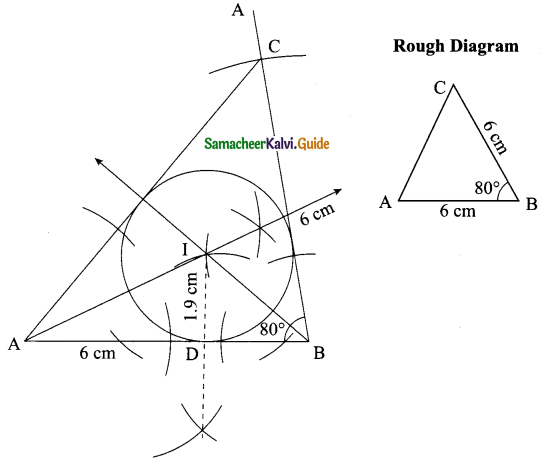Steps for construction:
Step 1: Draw the ΔABC with AB = 6 cm, ∠B = 80° and BC = 6 cm.
Step 2: Construct the angle bisectors of any two angles (A and B) and let them meet at I. Then I is the incentre of ΔABC.
Step 3: Draw perpendicular from I to any one of the side (AB) to meet AB at D.
Step 4: With I as centre and ID as radius draw the circle. This circle touches all the sides of the triangle internally.
Step 5: Measure of In-radius = 1.9 cm.